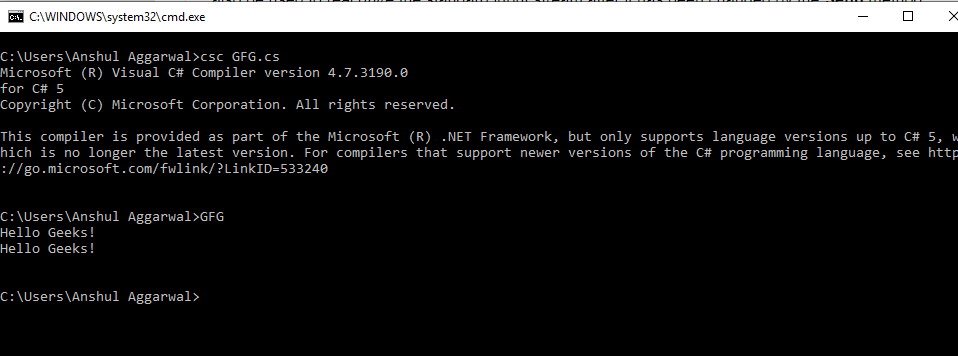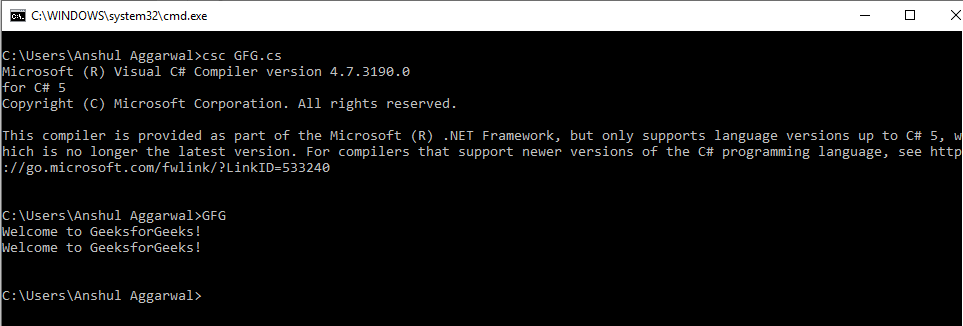Skip to content
Related Articles
Console.OpenStandardInput Method in C#
• Last Updated : 05 Mar, 2019

Console.OpenStandardInput Method is used to get the standard input stream. There are two overloads of OpenStandardInput method available in C# which are listed below:

• OpenStandardInput() Method
• OpenStandardInput(int32) Method

#### OpenStandardInput() Method

It is used to get the standard input stream. Make the object of Stream class and by using this method, the user can give the Input reference to that object. It creates a buffer which is used to take user input. This method can also be used to reacquire the standard input stream after it has been changed by the SetIn method.

Syntax:

`public static System.IO.Stream OpenStandardInput ();`

Example:

 `// C# program to illustrate the ``// OpenStandardInput() Method``using` `System;``using` `System.Text;``using` `System.IO;`` ` `class` `GFG {`` ` `    ``public` `static` `void` `Main()``    ``{`` ` `        ``// Stream Object declared and ``        ``// OpenStandardInput method is used``        ``Stream inputStream = Console.OpenStandardInput(); ``        ``byte``[] bytes = ``new` `byte``;``        ``int` `outputLength = inputStream.Read(bytes, 0, 50);``        ``char``[] chars = Encoding.UTF7.GetChars(bytes, 0, outputLength); ``        ``Console.WriteLine(``new` `string``(chars));``    ``}``}`

Output:#### OpenStandardInput(Int32) Method

It is also used to get the standard input stream which is set to specified buffer size. The value passed in this method determines the size of the buffer. This method can also be used to reacquire the standard input stream after it has been changed by the SetIn method.

Syntax: public static System.IO.Stream OpenStandardInput (int bufferSize);

Parameters:
buffersize: It is the internal stream buffer size.

Return Value: It returns the standard input stream.

Exception: This method will give ArgumentOutOfRangeException if the buffersize is less than or equal to zero.

 `// C# program to illustrate the ``// OpenStandardInput(Int32) Method``using` `System;``using` `System.Text;``using` `System.IO;`` ` `class` `GFG {`` ` `    ``// Main Method``    ``public` `static` `void` `Main()``    ``{`` ` `        ``// Using the Method``        ``Stream inputStream = Console.OpenStandardInput(100);``        ``byte``[] bytes = ``new` `byte``;``        ``int` `outputLength = inputStream.Read(bytes, 0, 100);``        ``char``[] chars = Encoding.UTF7.GetChars(bytes, 0, outputLength);``        ``Console.WriteLine(``new` `string``(chars));``    ``}``}`

Output:Reference:

My Personal Notes arrow_drop_up